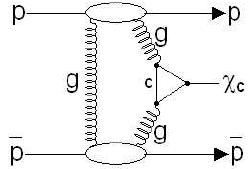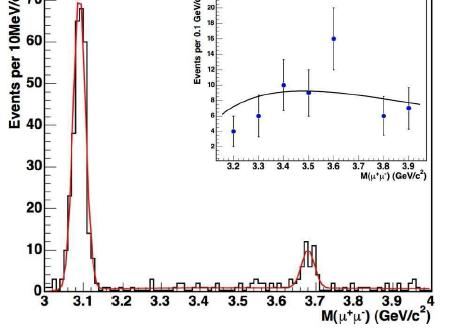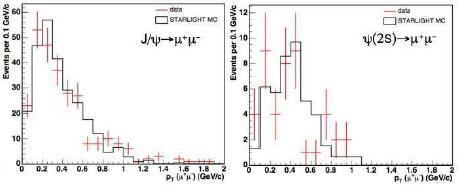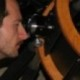## Three exquisite exclusive charmonium signals December 29, 2008

Posted by dorigo in news, physics, science.
Tags: , , ,

It is my pleasure today to report on an extremely interesting measurement recently produced by the CDF experiment, one which is dear to me for several reasons.

First of all, the measurement involves the study of charmonium -a fascinating system made by a charm-anticharm quark pair, the two quarks orbiting around each other for a tiny fraction of a second before annihilating. Charmonium -in the form of the$J/\psi$ particle- is the state of matter discovered in November 1974 by Nobel Laureates Samuel Ting (at Brookhaven) and Burton Richter (at SLAC), and which immediately confirmed the reality of quarks -entities whose reality had kept many brilliant minds skeptical during the previous decade. The$J/\psi$ and the other charmonium states have been since then a gold mine for the study of Quantum Chromodynamics, of B hadron phenomenology (through the easy-to-spot decays of the type$B \to J/\psi X$), and an invaluable calibration tool for particle trackers. Charmonium may one day be even used with profit to measure with high precision the top-quark mass at the LHC!

Second, the study focuses on what is called “exclusive production”, a spectacular signature at hadron colliders: it involves the production of the particle without the disintegration of the projectiles, through a colourless exchange. Basically, the charmonium meson appears out of nothing, and in the company of exactly nothing else. It is as if by a stroke of a magic wand, a spark is exchanged between a proton and an antiproton coming close together, and a single particle is created. Those of you who know what kind of a mess is routinely yielded by proton-antiproton collisions at 2 TeV of energy in the core of CDF and D0 cannot but join my awe for the very concept of clean single-body production.

Third, exclusive production processes are quite rare in hadron collisions, and they are extremely interesting because they may, one day, provide the means by which we can access a direct measurement of the quantum numbers of the Higgs boson at the LHC. Also, their theoretical calculation is not on very solid ground, so a measurement is of great help. This one by CDF is the first ever on exclusive charmonium in hadron-hadron collisions.

Some notes on the production mechanisms

Before I come to describe the measurement details, let me present you with two Feynman diagrams of the simplest reactions responsible for the exclusive creation of a charmonium system. As I said above, the exchange between proton and antiproton is colourless: this, while still being a QCD interaction! But how is it possible for quantum chromodynamics to mediate a colourless reaction ? We know that the carrier of the strong force is the gluon, a bi-coloured object. On the other hand, if a proton emits or absorbs a gluon, it will never keep intact: it will become coloured, and explode like a firework. Unless…Unless a second gluon immediately comes to the rescue, restoring the neutrality of the proton! This is what is needed for a strong interaction to produce a charmonium state: two gluons are emitted and absorbed by the proton and the antiproton, and the pair can thus leave the interaction point, slightly rattled but unharmed. The first pair of gluons meets halfways, creating a charm-quark loop. The loop then “closes” into a$\chi_c$ meson, a charmonium state with the right quantum numbers. This is the process shown on the right.

Notice that a two-gluon exchange cannot give rise to a$J/\psi$ meson. This is because of the invariance of strong interactions for the operation called “charge conjugation”. A discussion of the invariance properties of particle reactions under the operation of exchanging every particle with its antiparticle would take us too far, and is the proper subject of another post. I will just say here that the reaction is impossible: in quantum mechanics, one says that the “transition amplitude vanishes” for a two-gluon state converting into a vector particle.

Not so for the$\chi_c$ though, since that particle has zero angular momentum: it is not a vector, but a pseudoscalar meson. That is why we have examined$\chi_c$ production first. But exclusive$J/\psi$ production does occur anyway.

How, however, if we are to keep the two incoming baryons intact? Let us think at the problem from the point of view of the$J/\psi$ then. This particle is known to be quite slow to decay into hadrons. In fact, it has a lifetime much longer than similar hadronic states. So much so, in fact, that the very suppressed electromagnetic decay into electron or muon pairs gets a sizable share of the probability. An electromagnetic process competing with a strong process ? This is very weird: we know that the electromagnetic decay must have a rate proportional to the coupling constant$\alpha$, which is fifty times smaller than the strong coupling constant$\alpha_s$ for such low-energy phenomena. However, how is quantum chromodynamics going to disintegrate a$J/\psi$ ?

Phase one is simple: the charm and the anticharm get together in the same point of space, annihilating. Phase two is the emission of gluons: not a single one though, because the$J/\psi$ is colourless! And not just two, because of the quantum mechanical selection rule concerning C-parity we mentioned above! The minimum number of gluons is three. That means that we have to take the third power of$\alpha_s$ when computing the decay rate through QCD interactions, and so electromagnetic processes become competitive.

In reality, these three-gluon exchanges are even more suppressed than they ought to be. This is encoded in what is called “OZI rule”, by the name of Okubo, Zweig, and Iizuka: at any order of the strong coupling constant$\alpha_s$, “disconnected” strong interaction processes -ones that have no fermion lines propagating from the initial to the final state, but only gluons- occur with smaller frequency. This is a consequence of the variability of$\alpha_s$ with the energy of the gluon emission: in disconnected diagrams gluons have to carry an energy at least equal to the mass of the particles they are going to materialize in the final state, and the corresponding value of the coupling constant is therefore evaluated at a higher energy, and is thus much smaller than the one involved in the emission of softer gluons in non-disconnected diagrams. Confused ? Not a problem, forget the argument and let us return to$J/\psi$ production.

The above discussion just meant to show that we need three gluons to produce one$J/\psi$, since we now know that one such particle can decay to no less than three gluons. Now, let us take the protons -the projectiles producing the reaction- in the equation. Who is going to provide two gluons, and who is going to provide only one ? I can picture the proton and antiproton starting a bitter argument on this issue. The one who provides a single gluon is a dead baryon, since it will get its colourlessness unbalanced, and it will disgregate. This is bad, because the production process is not exclusive any longer if, together with a$J/\psi$ meson, we get additional hadrons.How then can we produce a vector charmonium particle in an exclusive fashion ? Electrodynamics comes to the rescue. One of the two projectiles yields a pair of gluons, and the other emits a photon. Both can remain intact this way, and angular momentum does not object. The process is shown on the right: as you see, the charm-anticharm pair becomes a colourless one after the second gluon is exchanged, and a$J/\psi$ (or a$\psi(2S)$ meson, which has the same properties except angular momentum) arises.

The CDF search

Now that we know how proton-antiproton collisions are expected to yield exclusive charmonium, we may look for it more meaningfully.

The search uses an integrated luminosity of 1.5 inverse femtobarns of proton-antiproton collisions, recorded by the CDF experiment during Run II. The trigger used to collect exclusive production candidates selects data with two muon tracks of opposite charge, with no energy recorded in the BSC1 system, a set of scintillation counters covering the rapidity intervals$5.4 < |\eta| < 5.9$. Such a requirement excludes data which may be similar kinematically to real exclusive production, but feature a few hadrons produced almost parallel to the proton-antiproton direction.

Offline, events are excluded if they contain any other detected particle but the two muons. However, the search for$\chi_c$ allows one electromagnetic energy cluster in the calorimeter, signalling the potential photon emitted along with two muons in the$\chi_c \to \mu^+ \mu^- \gamma$ decay.

After those cuts, the only potential background to address is cosmic-ray muons that cross the detector, leaving a long track that may be fit as a pair of opposite-charge muons if it crosses the detector in proximity of the beam. These events are rejected by requiring that the two muons have an azimuthal opening angle smaller than 3.0 radians, and rejecting ones that have timing information incompatible with the instant when the proton and antiproton bunches cross in the detector.

The invariant mass of the 402 events surviving the selection is histogrammed in the graph below, if it is in the 3.0 – 4.0 GeV range (ignore the inset, which shows a fit to the background). A clear peak of$J/\psi$ and$\psi(2S)$ candidates is apparent on a flat background of non-resonant QED dimuon pairs; the set of events in the$J/\psi$ peak which contain one additional photon candidate make the$\chi_c$ signal (65 events).The calculation of efficiencies is complicated by the fact that the exclusivity requirements remove bunch crossings which produced more than a single proton-antiproton interaction; this is a function of the number of particles in the bunches. After all is accounted properly, CDF can however determine the cross-section for charmonium production in exclusive processes with a good accuracy. The results are as follows:

•$\frac{d \sigma}{dy} (y=0) = 3.92 \pm 0.62 nb$ for$J/\psi$
•$\frac {d \sigma}{dy} (y=0) = 0.54 \pm 0.15 nb$ for$\psi(2S)$
•$\frac {d \sigma}{dy} (y=0) = 75 \pm 14 nb$ for$\chi_c$

The above results are in agreement with theoretical calculations, which have very large uncertainties. This measurement was long overdue: it is a very important input for theoretical calculations of exclusive processes.

The kinematical characteristics of the resulting$J/\psi$ and$\psi(2S)$ decay signals are studied by comparing data with a Monte Carlo simulation of photoproduction, finding good agreement. Below are shown the transverse momentum distributions of the resonances in the data (red points), compared to simulation (black histograms).In conclusion, I am happy to see these very intriguing signals appear in CDF data, and I congratulate with the main authors of the analysis, Long Zhang, Mike Albrow, and James Pinfold! You can find more information on the analysis in the public web page of the result.1. carlbrannen - December 30, 2008

Nice bumps. It seems to me that you could change the gluon number problem by using a ggg interaction, aren’t they allowed?

The paper I’m planning on sending to Phys Math Central revolves around the heavy quarkonium states. In short, it says that the six J/psi mesons and the six Upsilon mesons are in direct correspondence with each other, and also with the six leptons; they are corresponding excitations.

To see the correspondence, you have to split the sixes up into two triplets each, with formulas similar to each other and to the charged leptons and the neutrinos. Let’s see if I can write the mass formulas here using the local LaTeX. Since there is no preview, this is an exercise in perfection. Which reminds me, the heavy snow in Seattle has kept me from the chess club for 2 weeks but now the roads are clear.

Then the three charged leptons have masses (in MeV) given by$\sqrt{m_{eg}} = 25.054 309 435(\sqrt{2}+\cos(2/9 + 2g\pi/3)),$
where g is the generation number. The above constant was chosen so that it gives the masses to within experimental error. The three neutrino masses (in eV) are given by$\sqrt{m_{\nu g}} = 0.1414(\sqrt{2}+\cos(2/9 + 2g\pi/3 + \pi/12)).$
This gives the right values for the neutrino oscillation measurements, which are of the absolute values of the differences in the squares.

Thus the leptons can be written as two slightly different triplets. The claim is that the J/psi and Upsilon also show the same triplets. Here’s the J/psi mass formulas (using the same overall constant$\mu = 25.054 ...$, as in the charged lepton formula):$\sqrt{m_{\psi 1 g}} = \mu(2.44247 - 0.25002\cos(2/9+2g\pi/3+\pi/12)),$$\sqrt{m_{\psi 2 g}} = \mu(2.51049 - 0.08943\cos(2/9+2g\pi/3)),$
and for the Upsilon:$\sqrt{m_{\Upsilon 1 g}} = \mu(3.99332 - 0.12667\cos(2/9+2g\pi/3+\pi/12)),$$\sqrt{m_{\Upsilon 2 g}} = \mu(4.13723 - 0.07754\cos(2/9+2g\pi/3)).$
Note that each equation uses two arbitrary constants to give three masses. And some of the arbitrary constants are suggestively close to rational numbers like 4 or 1/4.

Unlike the leptons, the heavy meson fits are not exact, but have errors of magnitude around the isospin splitting (which ought to be how inaccurate a color calculation should be). For the J/psi you get
[3096.916, 3775.2, 4421.1] versus experiment
[3096.916(.011), 3775.2(1.7), 4421(4)]
and
[3686.08, 4040, 4150] versus
[3686.093(.034), 4039(1), 4153(3)].

The Upsilon mesons
[9456, 10035, 10554] versus
[9460.30(26), 10023.26(31), 10579.4(1.2)]
and
[(10355.2, 10864.4, 11019.5] versus
[10355.2(.5), 10865(8), 11019(8)].
Note that the really bad fit is that of the lighter Upsilon mesons. It may not be a coincidence that these are the states that are most easily modeled using non relativistic QM and have now been renamed$\Upsilon(1S), \Upsilon(2S), \Upsilon(4S)$ if I recall.

Anyway, I am still busily improving the paper. Phys Math Central requires a background section to familiarize non specialists which is a very good idea.2. Namit - December 30, 2008

Dear Tomasso,
Could you provide a pointer/link to the theory paper with which the results are compared with.
Regards3. dorigo - December 30, 2008

Hi Carl,

good to know you are working hard. I have sent you the voucher, you should receive it in a week or so. The coincidences you mention seem striking to me, but I think one would have to complement such an analysis with an estimate of the “trial factor” of putting together constants and functions to fit those numbers. That may give the reader some perspective.

Namit, the link I had provided was not html’ed correctly, now I have fixed it. If you visit the web site of the analysis you will find more information. In any case I paste below a few theoretical references:

A. Szczurek, Proc. Blois 2007 (Hamburg), 231, arXiv:0709.0224.
S. Klein and J. Nystrand, Phys. Rev. Lett. 92:142003 (2004).
V.P. Goncalves and M.V.T. Machado, Eur. Phys. J. C40, 519 (2005).
L. Motyka and G. Watt, arXiv:0805.2113.
F. Yuan, Phys. Lett. B510, 155 (2001).

Cheers,
T.4. Igor Ivanov - December 30, 2008

Dear Namit,

the field these results belong to is called “diffraction at hadronic colliders”. Tommaso cited a few theory papers with some particular examples of calculations. There is in fact a series of workshops devoted to diffraction, and the last one, Diffraction-2008, took place last September, the website is http://www.cs.infn.it/diff2008/
There you can find many more results and theoretical calculations.

There is also a project for the LHC, called FP420, which aims at detecting both protons with very forward roman pots (220+420 meters away from the main detector), which together with the information from central detector will make a very clean study of such processes possible at the LHC.

Cheers,
Igor5. Carl Brannen - December 30, 2008

Tommaso,

I’ve been very busy on this and want to do it right. There are a total of 38 hadron equations like the ones for heavy quarkonium, but most hadron resonance masses are not known to great accuracy so it is only in heavy quarkonium where the fits are much worse than the experimental result. So I did the statistics for heavy quarkonium fits only.

In the process of doing the Montecarlo for random ratios I found a result that surprised me a little. Take three samples from a uniformly distributed random variable. Rearrange the three samples so that a<b<c. Then what is the distribution of the ratio:
(b-a)/(c-a)? Clearly the ratio is between 0 and 1. To calculate it from first principles, the rearrangement is most of the trouble as the inequalities break up the triple integral from 0 to 1. I found the result by simulation and then proved it because it’s easier if you already know the answer…

Ratios are useful for analyzing structure in data that is organized in triplets. For example, if the mass formula were like that for hydrogen like atoms (i.e. nuceli plus one electron):
M_n = M – k/n^2
for k a constant and M the sum of the nuclei and electron masses, then the ratio (b-a)/(c-a) for the three lowest masses, n=1,2,3 is always 27/32.6. carlbrannen - December 30, 2008

Tommaso,

I did a Monte Carlo to find the distributions and learned some things about ratios of random variables doing this. A little puzzle that an experimentalist might enjoy:

Pick three numbers from a uniform [0,1] distribution (or any other uniform distribution, same result). Rearrange them so that they are ordered a<b<c. Then the ratio (b-a)/(c-a) has what distribution?

With 6 masses, there are 20 ways of splitting them up into two distinct groups of three so the best fit is far better. The simulation is easy. I use java.

By the way, the easiest way to characterize a triplet of three numbers a<b<c is by that ratio (b-a)/(c-a). For example, if the three numbers happen to be the masses of the three lowest excitations of a hydrogen-like atom (i.e. some atom stripped to one electron), then they follow a law$E_n = s - t/n^2$ where s and t are constants. Uh, s is the mass of the bare nucleus plus the mass of the electron. For such a triplet, we have:
(b-a)/(c-a) = 27/32.
Looking at ratios is a good way of picking triplets out of data.

I did the distribution calculations for heavy quarkonium only, as it is unique in having the masses so accuratelyl measured that the fits are worse than the experimental measurements. This makes the calculation easy. The paper includes 36 mass formlas for other hadrons (i.e 120 counting heavy quarkonium). Also there are some heavy mesons whose masses are accurately known but not the quantum numbers and the paper predicts the quantum numbers for them.7. Michael Schmitt - December 31, 2008

Hi Tommaso, very nice post!

I like this analysis as well. For me, the differences between the production mechanism for the vector mesons (J/psi and Psi(2s)) and the scalar (chi_c) are very important. As you alluded to, the chi_c is a stand-in for the Higgs boson. For the chi_c, the charm quark plays the main role, while for the Higgs boson, it is the top quark. If I understand correctly, the CDF results confirm the moderate-upper end of the theoretical predictions for exclusive Higgs production at the LHC. Mike Albrow wrote a superb exposition of this physics – see arXiv:0812.0612

regards,
Michael8. Namit - December 31, 2008

Thanks a lot Tommaso and Igor for the links and references.9. Louise - December 31, 2008

HI Tommaso: Off-subject, but I would like to draw your attention to the blog of our friend Kea. She has a new job in Oxford, and was due to speak there January 7. The authorities have taken her passport until further notice and left her to wash dishes. You may recall my experiencing similiar problems with UK immigration. This is madness.

Sorry comments are closed for this entry